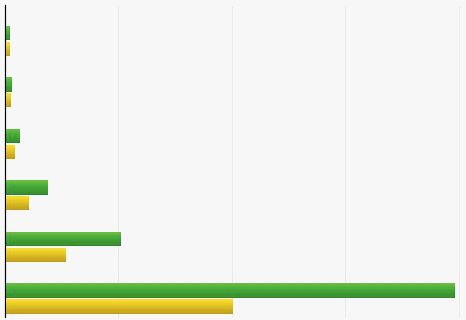微信公众号 关注我们 知识星球关注 @泰晓科技与数百位一线 Linux 工程师做朋友，您准备好了吗？分享 Linux 产品实战经验。 与业界 Linux 专家交流。# IP 校验和计算优化：四两拨千斤

 微信公众号 知识星球关注 @泰晓科技与数百位一线 Linux 工程师做朋友，您准备好了吗？分享 Linux 产品实战经验。 与业界 Linux 专家交流。

by Chen Jie of TinyLab.org 2014/09/05

## 前言## 阅读：IP校验和的实现

arch/mips/lib/csum_partial.S 优化实现了相关函数：

• `uint32_t csum_partial(const void *buffer, int len, uint32_t sum)`

对应 C 实现在 lib/checksum.c。计算当前 buffer 的校验和，结果并入传入的校验和（参数sum）。这是说我们可以将一个大 buffer，分片断来计算校验和。参数 len 必须是偶数，除非是最后一个片断。

• `uint32_t csum_partial_copy_from_user(const void __user *src, void *dst, int len, uint32_t sum, int *csum_err)`

对应C实现在 lib/checksum.c。该函数边拷贝，边计算校验和。其中拷贝的源地址可能位于用户空间，因此需要关照访存错误的情况（因此有返回参数 csum_err 来指明错误）。

在MIPS实现中，该函数实际位于 arch/mips/include/asm/checksum.h，此处做了一个简单封装 —— `源地址在用户空间？__csum_partial_copy_from_user ：__csum_partial_copy_kernel` —— `csum_partial.S` 优化了实际调用的后两函数。

• `csum_partial` 核心工作为:

``````LOAD    temp, (src) # 装载到temp（某个寄存器）
ADDC(sum, temp)         # 结果保存到sum（某个寄存器）
``````
• `csum_partial_copy*` 核心工作为：

``````LOAD    temp, (src)
ADDC(sum, temp)
STORE   temp, (dst) # 附带拷贝
``````
• ADDC 是个宏，展开成一组计算操作：

``````#define ADDC(sum, temp1) \
ADD sum, temp1 \
sltu    temp2, sum, temp1 /* sum值是否比加数来的小，如是则溢出了(此时temp2被置1) */ \
ADD sum, temp2
``````

• `csum_partial` 的优化实现，大体是按照逐级“升级”对齐条件的方法：

Step1:   半字（16位）对齐 ? 下一个 : 升级到半字对齐（升级方法：处理一字节数据）

Step2:   字（32位）对齐 ? 下一个 : 升级到字对齐（升级方法：处理半字数据）

一直到8字（256位，32字节）对齐，之后循环按块处理。（P.S. MIPS通用访存宽度为64位，即双字，为啥对齐条件要一直升到8字呢？一是方便替换成访存宽度更宽的指令，例如我们可以很方便地替换成龙芯128位访存指令gslq/gssq；另一方面大概是考虑缓存/数据预取的隐性对齐要求）

• `csum_partial_copy*` 的优化实现

抄/改自 memcpy 优化实现，即用左右部装载/存储指令，将目的地址（dst）升至64位对齐，然后依据源地址（src）对齐状况，两分支取一（左右部装载 vs 直接8字节装载）。

## Tunning

``````sum = A + B >= UINT64_MAX+1 ? A+B-(UINT64_MAX+1)+1 : A+B
``````

``````ADDC(sum, A)
ADDC(sum, B)
ADDC(sum, C)
ADDC(sum, D)
``````

• 你办的这件事所需材料齐备
• 并且办事窗口空闲

``````1   LOAD    A, (src)
2   LOAD    B, 8(src)
3   LOAD    C, 16(src)
4   LOAD    D, 24(src)
5   ADDC(sum, A)
6   ADDC(sum, B)
7   ADDC(sum, C)
8   ADDC(sum, D)
``````

``````1   ADDC(A, B)
2   ADDC(sum, A)
3   ADDC(C, D)
4   ADDC(sum, C)
``````

## 验证

• ADDC(A, B)记为“A B”
• 于是A ** B** = A + B >= @ ? A+B-@+1 : A+B
• 64位无符号表达上限记为“@”，即@等于“2^64”
• 对于参与运算的数A，其区间在[0, @-1]，即 `uint64_t` 所表示范围。

• 加法1溢出，加法2不溢出。
• 加法1不溢出，加法2溢出。
• 加法1和加法2均溢出。
• 加法1和加法2均不溢出。

### 情况1：加法1溢出，加法2不溢出

``````1.1   sum + A >= @
1.2   sum + A - @ + 1 + B < @
sum 烫 A 烫 B = sum + A + B - @ + 1
``````

``````2   => A 烫 B = A + B - @ + 1

=> sum 烫 (A 烫 B) = sum + A + B - @ + 1  # (1.2)
``````

``````3   => A 烫 B = A + B

4   sum + A + B >= @ + B >= @   # (1.1) 两边加B
=> sum 烫 (A 烫 B) = sum + A + B - @ + 1
``````

### 情况2：加法1不溢出，加法2溢出

``````1.1   sum + A < @
1.2   sum + A + B >= @
sum 烫 A 烫 B = sum + A + B - @ + 1
``````

``````2   => A 烫 B = A + B - @ + 1

3   sum + A + B - @ + 1 < @ + B - @ + 1 # (1.1) 两边加B-@+1
4   => sum + A + B - @ + 1 < B + 1 <= @
5   => sum + A + B - @ + 1 < @
=> sum 烫 (A 烫 B) = sum + A + B - @ + 1
``````

``````2   => A 烫 B = A + B

=> sum 烫 (A 烫 B) = sum + A + B - @ + 1  #(1.2)
``````

### 情况3：加法1和加法2均溢出

``````1.1   sum + A >= @
1.2   sum + A + B - @ + 1 >= @
sum 烫 A 烫 B = sum + A + B - 2@ + 2

2   A + B >= 2@ - 1 - sum   # (1.2) 移项得
3   已知1 + sum <= @      # 0 <= sum <= @-1
4   => @ <= @ - 1 - sum + @
5   => @ <= 2@ - 1 - sum
6   => @ <= 2@ - 1 - sum <= A + B   # (2)
7   => A + B >= @       # (6) 整理得
8   => A 烫 B = A + B - @ + 1

=> sum 烫 (A 烫 B) = sum + A + B - 2@ + 2 # (1.2)
``````

### 情况4：加法1和加法2均不溢出

``````1.1   sum + A < @
1.2   sum + A + B < @
sum 烫 A 烫 B = sum + A + B

2   A + B < @ - sum <= @    # (1.2) 移项得, sum >= 0
3   => A + B < @
4   => A 烫 B = A + B

=> sum 烫 (A 烫 B) = sum + A + B  #(1.2)
``````